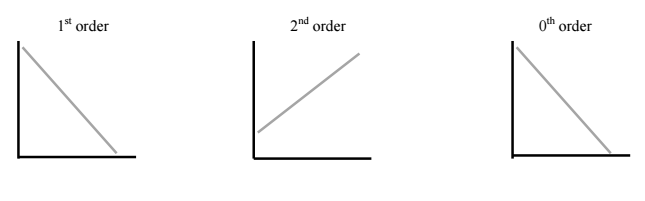Chemistry Practice Problems Identifying Reaction Order Practice Problems Solution: Fill in plots for the following reaction orders. B...

# Solution: Fill in plots for the following reaction orders. Be sure to label as much as possible (axes, slopes, intercepts).

###### Problem

Fill in plots for the following reaction orders. Be sure to label as much as possible (axes, slopes, intercepts).Identifying Reaction Order

Identifying Reaction Order

#### Q. Fluorine-18 undergoes positron emission with a half life of 1.10x 10  2 minutes. If a patient is given a 248 mg dose for a PET scan, how long will it ...

Solved • Wed Mar 16 2016 18:11:18 GMT-0400 (EDT)

Identifying Reaction Order

#### Q. A reaction has a rate constant of 0.0185 s-1 and the only reactant has an initial concentration of 0.135 M. What is the concentration in molarity of t...

Solved • Sun Feb 21 2016 11:17:49 GMT-0500 (EST)

Identifying Reaction Order

#### Q. Dinitrogen pentoxide (N2O5) decomposes to NO2 and O2 at relatively low temperatures in the following reaction: 2N2O5(aq) → 4NO2(aq) + O2(g) This rea...

Solved • Sun Feb 21 2016 11:11:23 GMT-0500 (EST)

Identifying Reaction Order

#### Q. For what order reaction does the half-life get longer as the initial concentration increases? A. zeroth order B. first order C. second order D. no...

Solved • Sun Feb 21 2016 11:04:58 GMT-0500 (EST)